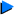# Miscellaneous ProblemsFind the remainder when 3x3 - x2 + 7x + 5 is divided by 3x + 2.Given that 2x3 - x2 - 2x + 3 = (Ax + B)(x - 1)(x + 2) + C(x - 1) + D, find the values of A, B, C and D. Hence or otherwise, deduce the remainder when

2x3 - x2 - 2x + 3 is divided by x2 + x - 2.Solve the equation 2x3 + x2 - 19x = 6, giving your answers to two decimal places where necessary.The expression x3 + px2 + qx + 6 has the same remainder when divided by

x + 1 and 2 - x. Given that the remainder when the expression is divided by x + 3 is -60, find the value of p and of q.

remainder = f(-2/3)
= 3(-2/3)3 - (-2/3)2 + 7(-2/3) + 5
= -1
2 - 1 - 2 + 3 = D
D = 2
Let x = -2
-16 - 4 + 4 + 3 = -3C + 2
3C = 15
C = 5
Let x = 0
3 = B (-1)(2) -5 + 2
-2B = 6
B = -3
Sub any value other than 1, 2 & 0 into x
A = 2
2x3 - x2 - 2x + 3 (Ax + B)(x - 1)(x + 2) + C(x - 1) + D
Since x2 + x - 2 = (x - 1)(x + 2), (note the similarity?)
thus, remainder = C(x - 1) + D
= 5(x - 1) + 2
= 5x - 3

let f(x) = 2x3 + x2 - 19x - 6
(x - 3) f(3) = 0(x - 3) is a factor of f(x)
let f(x) = (x - 3)(Ax2 + Bx + C)
A = 2
C = 2
let x = 1
2 + 1 - 19 - 6 = -2(2 + B + 2)
-22 = -8 - 2B
B = 7f(x) = (x - 3)(2x2 + 7x + 2)
2x2 + 7x + 2 = 0
x =
= -3.19 or -0.31 (3 s.f.)x = -3.19 or -0.31 or 3
by remainder theorem, f(-1) = f(2)
-1 + p - q + 6 = 8 + 4p + 2q + 6
p - q - 5 = 4p + 2q + 14
3p + 3q = 9 --------(1)
by remainder theorem, f(-3) = -60
-27 + 9p - 3q + 6 = -60
3q = 39 + 9p --------(2)
sub (2) into (1):
3p + 39 + 9p = -9
12p = -48
p = -4
q = 1